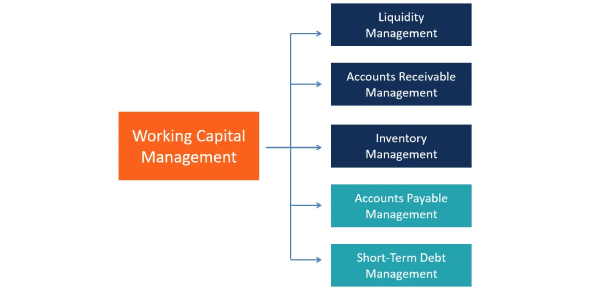# Working Capital Management MCQ Quiz

10 Questions | Attempts: 10356
ShareSettingsHow well do you understand working capital management? Here is an interesting 'working capital management' quiz. Take this quiz to see how strong your knowledge of the accounts subject you have. Working capital management is one of the very important components of financial management. This subject is concerned with the short-term finance of the business, which is a closely related trade between profitability and liquidity. If you are prepared to take this test, let's begin then. All the best for a perfect score!

• 1.
Which of the following would not be financed from working capital?
• A.

Cash float.

• B.

Accounts receivable.

• C.

Credit sales.

• D.

A new personal computer for the office.

• 2.
Which of the following defines the difference between the current ratio and the quick ratio?
• A.

The current ratio involves inventories, and the quick ratio does not.

• B.

The current ratio does not involve inventories, and the quick ratio does.

• C.

The current ratio involves physical capital, and the quick ratio does not.

• D.

The current ratio does not involve physical capital, and the quick ratio does.

• 3.
Which of the following options is not true for the matching strategy?
• A.

All assets must be financed with permanent long-term capital.

• B.

Temporary current assets must be financed with temporary working capital.

• C.

Permanent current assets must be financed with permanent working capital.

• D.

Long-term assets must be financed from long-term capital.

• 4.
Which among the following working capital strategies is the most aggressive?
• A.

Making greater use of short-term finance and maximizing net short-term assets.

• B.

Making greater use of long-term finance and minimizing net short-term assets.

• C.

Making greater use of short-term finance and minimizing net short-term assets.

• D.

Making greater use of long-term finance and maximizing net short-term assets.

• 5.
Which of these metrics is not used for measuring the length of the cash cycle?
• A.

Acid test days.

• B.

Accounts receivable days.

• C.

Accounts payable days.

• D.

Inventory days.

• 6.
Which of these are considered components of working capital management?
• A.

Inventories

• B.

Current liabilities

• C.

Accounts receivable

• D.

Accounts payable

• E.

Cash

• 7.
Which of these is the correct formula for calculating working capital?
• A.

Working capital = current assets – current liabilities.

• B.

Working capital = current assets + current liabilities.

• C.

Working capital = current assets - inventory - accounts payable.

• D.

None of the above

• 8.
The other name for working capital is _______________.
• A.

Operating capital

• B.

Current assets capital

• C.

Capital relating to the main projects of the company

• D.

None of the above

• 9.
Which of these methods is NOT used for calculating the working capital cycle?
• A.

Percentage of sales method

• B.

Operating cycle approach

• C.

Trial and error method

• D.

None of the above

• 10.
What are current assets?
• A.

The assets that can be sold easily by the companies

• B.

The assets that are converted into cash within a period of one year

• C.

Assets that are held by the companies to pay off the current liabilities

• D.

All of the above

## Related TopicsBack to top
×

Wait!
Here's an interesting quiz for you.